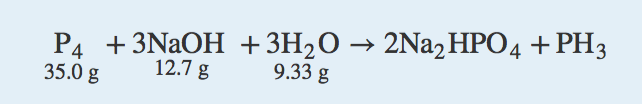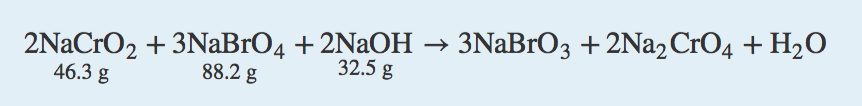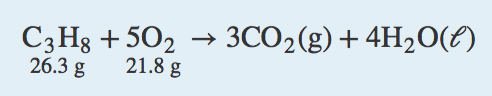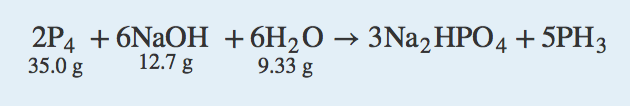Chapter 5. Stoichiometry and the Mole

# End-of-Chapter Material

### Additional Exercises

1. How many molecules of O2 will react with 6.022 × 1023 molecules of H2 to make water? The reaction is 2 H2(g) + O2(g) → 2 H2O(ℓ).

2. How many molecules of H2 will react with 6.022 × 1023 molecules of N2 to make ammonia? The reaction is N2(g) + 3 H2(g) → 2 NH3(g).

3. How many moles are present in 6.411 kg of CO2? How many molecules is this?

4. How many moles are present in 2.998 mg of SCl4? How many molecules is this?

5. What is the mass in milligrams of 7.22 × 1020 molecules of CO2?

6. What is the mass in kilograms of 3.408 × 1025 molecules of SiS2?

7. What is the mass in grams of 1 molecule of H2O?

8. What is the mass in grams of 1 atom of Al?

9. What is the volume of 3.44 mol of Ga if the density of Ga is 6.08 g/mL?

10. What is the volume of 0.662 mol of He if the density of He is 0.1785 g/L?

11. For the chemical reaction

2 C4H10(g) + 13 O2(g) → 8 CO2(g) + 10 H2O(ℓ)

assume that 13.4 g of C4H10 reacts completely to products. The density of CO2 is 1.96 g/L. What volume in liters of CO2 is produced?

12. For the chemical reaction

2 GaCl3(s) + 3 H2(g) → 2 Ga(ℓ) + 6 HCl(g)

if 223 g of GaCl3 reacts completely to products and the density of Ga is 6.08 g/mL, what volume in milliliters of Ga is produced?

13. Calculate the mass of each product when 100.0 g of CuCl react according to the reaction

2 CuCl(aq) → CuCl2(aq) + Cu(s)

What do you notice about the sum of the masses of the products? What concept is being illustrated here?

14. Calculate the mass of each product when 500.0 g of SnCl2 react according to the reaction

2 SnCl2(aq) → SnCl4(aq) + Sn(s)

What do you notice about the sum of the masses of the products? What concept is being illustrated here?

15. What mass of CO2 is produced from the combustion of 1 gal of gasoline? The chemical formula of gasoline can be approximated as C8H18. Assume that there are 2,801 g of gasoline per gallon.

16. What mass of H2O is produced from the combustion of 1 gal of gasoline? The chemical formula of gasoline can be approximated as C8H18. Assume that there are 2,801 g of gasoline per gallon.

17. A chemical reaction has a theoretical yield of 19.98 g and a percent yield of 88.40%. What is the actual yield?

18. A chemical reaction has an actual yield of 19.98 g and a percent yield of 88.40%. What is the theoretical yield?

19. Given the initial amounts listed, what is the limiting reagent, and how much of the other reactants are in excess?20. Given the initial amounts listed, what is the limiting reagent, and how much of the other reactants are in excess?21. Verify that it does not matter which product you use to predict the limiting reagent by using both products in this combustion reaction to determine the limiting reagent and the amount of the reactant in excess. Initial amounts of each reactant are given.22. Just in case you suspect Exercise 21 is rigged, do it for another chemical reaction and verify that it does not matter which product you use to predict the limiting reagent by using both products in this combustion reaction to determine the limiting reagent and the amount of the reactant in excess. Initial amounts of each reactant are given.### Answers

1.

1.2044 × 1024 molecules

3.

145.7 mol; 8.77 × 1025 molecules

5.

52.8 mg

7.

2.99 × 10−23 g

9.

39.4 mL

11.

20.7 L

13.

67.91 g of CuCl2; 32.09 g of Cu. The two masses add to 100.0 g, the initial amount of starting material, demonstrating the law of conservation of matter.

15.

8,632 g

17.

17.66 g

19.

The limiting reagent is NaOH; 21.9 g of P4 and 3.61 g of H2O are left over.

21.

Both products predict that O2 is the limiting reagent; 20.3 g of C3H8 are left over.

## LicenseEnd-of-Chapter Material by Jessie A. Key is licensed under a Creative Commons Attribution-NonCommercial-ShareAlike 4.0 International License, except where otherwise noted.